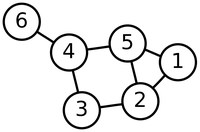# Glossary

## Degree

The degree of a node is the number of edges that are incident to the node. For example, consider the graph below. The degree of node 6 is 1, the degree of nodes 1 and 3 is 2, and the degree of nodes 2, 4, and 5 is 3.